Date: Sun, 15 Nov 1998 20:27:51 -0800 Sender: sara To: QandQ@MathCentral.uregina.ca Subject: middle 6-9 Hello! I am wondering why 16 and multiples of 16 do not work in the ancient duplation method of multiplication used by the Egyptians. I discovered the method in the Journeys math text gr 7 level. Just curious. Am I doing something wrong?? thanks Sara teacher Dear Sara The doubling method should work for any numbers since it is based on Algebra. Let's look at the theory to see why the doubling method works. We want to know the answer to 16xa where a represents any number. By the doubling method we write: 1xa = 1a, 2xa = 2x1a = 2a, 4xa = 2x2a = 4a, 8xa = 2x4a = 8a, 16xa = 2x8a = 16a.   At this point you have the answer to 16xa and need to go no further. But if you say that you want to use the technique shown on page 45 of Journeys in Mathematics 7, LeSage et al, Prentice Hall Ginn then you could could combine: 8xa, 4xa, 2xa, 1xa and 1xa or 8xa, 4xa, 2xa and 2xa or etc. In fact what you have discovered is that, when you are multiplying by powers of 2, the answer appears directly but that you can also obtain the answer by combining all of the answers from the previous powers of 2 and then adding again the 1st answer obtained by multiplying the number by 1. i.e.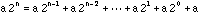Dividing both sides of the previous equation by a and subtraction 1 from both sides gives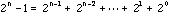This can be proved as follows.   Write the right side of the previous expression as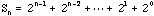Multiply both sides by 2 to get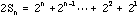Subtracting the previous two equations gives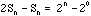or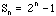Jack is one of the authors of JIM 7 and wants thank you for this insight.   Another way to look at what is going on with the duplation method of multiplication is to notice that the doubling procedure expresses the multiplyer in base 2. So if you want to find, say 19a, again by the doubling method write 1xa = 1a, 2xa = 2x1a = 2a, 4xa = 2x2a = 4a, 8xa = 2x4a = 8a, 16xa = 2x8a = 16a. Three of the rows are highlighted since 19 = 16 + 2 + 1. This expresses 19 in base 2 as 19 = 10011(base 2). Hence if your multiplyer is 16 you only need to read off the fifth row since 16 = 10000(base 2). Cheers, Jack and Harley Go to Math Central To return to the previous page use your browser's back button.